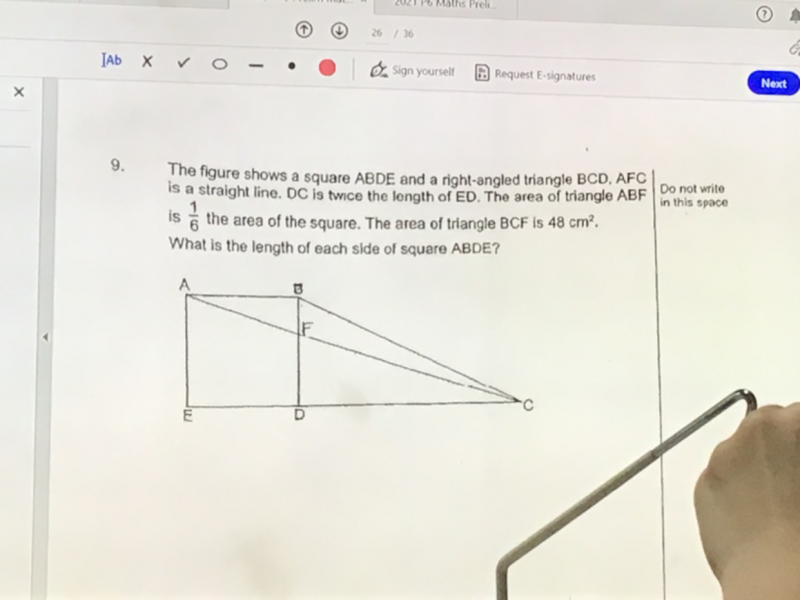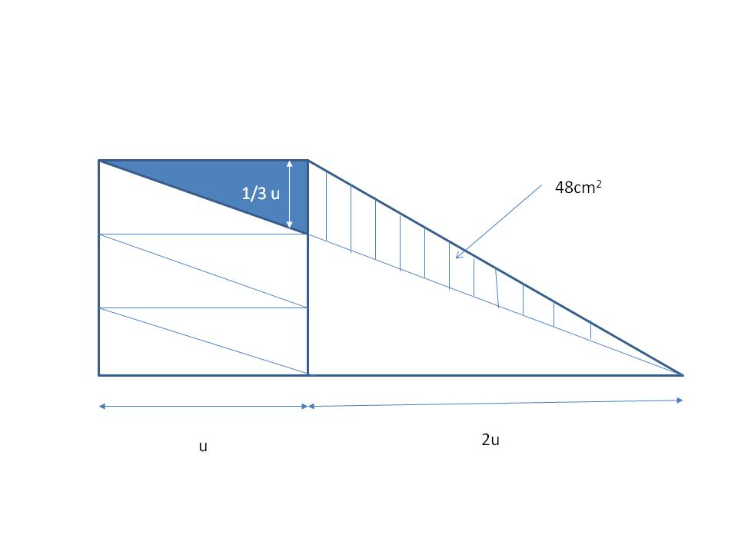# QuestionPls help. ThxFrom the diagram, using area of triangle  1/2 b x h on the triangle on the right with stripes,

(1/2)(1/3)u (2u) = 48

1/3 u2 = 48

u2  = 48 x 3 = 144

u = 12

Hence the length of each side of square is 12cm.

0 Replies 2 Likes